• 西安邮电学院 实 验 报 告 实验名称 形态学图像处理 课程名称 数字图像处理A 姓名 成绩 班级 学号 日期 2011.5.31 地点 3#531 备注基于matlab实现形态学图像处理 1. 对两幅图像进行腐蚀膨胀处理显示处理前后图像 2. ...
• 1、对二值图像进行形态学运算。2、对二值图像进行边缘提取。
• 实验形态学图像处理


实验五 形态学图像处理
1 实验目的2 实验环境3 实验内容4 实验心得

1 实验目的
1、了解形态学的基本理论和方法。 2、掌握对图像进行膨胀、腐蚀的方法。 3、掌握开闭运算。
2 实验环境
matlab
3 实验内容

1、采用形态学处理方法实现图像去噪。

%（1）lab51.m
close all;
clear;
clc;
I=rgb2gray(I);
I=im2bw(I);
I=double(I);

J1=imnoise(I,'salt & pepper',0.02); %椒盐噪声

se=strel('disk',1);
% se=[0 1 0;1 1 1;0 1 0];
se.Neighborhood

%去掉黑噪声
J2=imdilate(J1,se);
J2=imerode(J2,se);
%二值反转，去掉白噪声
J3=imcomplement(J2);
% J2=dilation(J2,se);
% J2=erosion(J2,se);
J3=dilation(J3,se.Neighborhood);
J3=erosion(J3,se.Neighborhood);
%二值反转
J4=imcomplement(J3);

figure('NumberTitle','off','name','形态学处理去噪'),
subplot(2,3,1);imshow(I);title('原始图像','FontName','宋体');
subplot(2,3,2);imshow(J1);title('添加噪声','FontName','宋体');
subplot(2,3,4);imshow(J2);title('去除黑噪声','FontName','宋体');
subplot(2,3,5);imshow(J3);title('去除白噪声','FontName','宋体');
subplot(2,3,6);imshow(J4);title('去噪后的图像','FontName','宋体');

%（2）自定义图像膨胀函数dilation.m
function j=dilation(i,se)

[w,h]=size(se);
[width,height]=size(i);

%  j=zeros(width,height);
j=i;

for row=1:width-(w-1)
for col=1:height-(h-1)
mark=i(row:row+(w-1),col:col+(h-1));
temp=se.*mark;
if sum(temp(:))>0 %只要卷积结果中有非零元素，则将输出图像的对应位置赋值1
j(row+floor((w-1)/2),col+floor((h-1)/2))=1;
else
j(row+floor((w-1)/2),col+floor((h-1)/2))=0;
end
end
end

%（3）自定义图像腐蚀函数erosion.m
function j=erosion(i,se)

[w,h]=size(se);
[width,height]=size(i);
j=zeros(width,height);

count_1=0;
for x=1:w
for y=1:h
if se(x,y)==1
count_1=count_1+1;
end
end
end

for row=1:width-(w-1)
for col=1:height-(h-1)
mark=i(row:row+(w-1),col:col+(h-1));
temp=mark.*se;
count=0;
for x=1:w
for y=1:h
if temp(x,y)~=0
count=count+1;
end
end
end
%比较结构元素与c中的1的数量，如果一样多，则该点的值为1
if count==count_1
j(row+floor((w-1)/2),col+floor((h-1)/2))=1;
else
j(row+floor((w-1)/2),col+floor((h-1)/2))=0;
end
end
end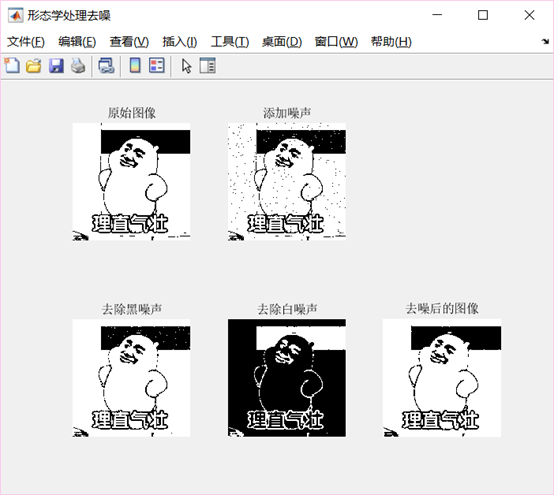2、对图像分割实验（实验四）得到的分割结果进行形态学处理，对肺部区域的空洞进行填充。

%主函数lab52.m
close all;
clear;
clc;

se=strel('disk',7);
se.Neighborhood

J1=dilation(I,se.Neighborhood);
J1=erosion(J1,se.Neighborhood);
figure('NumberTitle','off','name','形态学处理去噪'),
subplot(1,2,1);imshow(I);title('原始图像','FontName','宋体');
subplot(1,2,2);imshow(J1);title('填补空洞','FontName','宋体');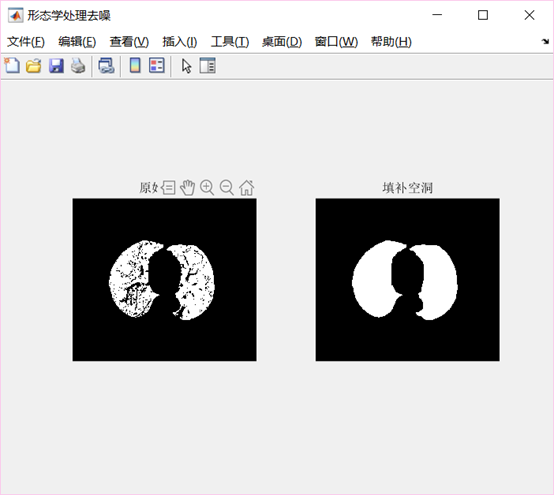4 实验心得
进一步理解了形态学处理图像的方法，实现了图像的膨胀、腐蚀以及开闭运算等操作。实验中没有直接自定义开闭运算函数，在操作过程中，使用同一结构元素对图像先膨胀后腐蚀，相当于闭运算
展开全文matlab 数字图像处理
• 针对基于数学形态学的去除高浓度椒盐噪声不足问题，采用了一种基于数学形态学的噪声去除方法。所提滤波方法分为预滤波和滤波两个阶段。在预滤波阶段，设定一个简单的检测算子用于构造逐点自适应的3×3像素大小结构...
• 因此，本文以数字图像为载体，研究基于形态学的图像分割技术，并进行物体个数计算应用和车道线检测的应用。对图像中物体个数的计算以及车道线检测应用，不仅需要对采集到的图像进行预处理，而且要针对特定的目的进行...
• 针对现有模糊聚类分割算法对噪声的鲁棒性差且提取的图像特征不充分等问题, 本文提出了一种结合形态学重建和超像素的多特征模糊 C-均值(FCM)分割算法. 首先, 利用形态学闭合重建处理原图像, 提高了算法的鲁棒性和细节...
• 形态学滤波算法的提出,在很大程度上提高了雷达点云的滤波效果。准确高效地分离地面点和地物点,是...为了检验等值线-形态学滤波算法的效率和精度,将其与渐进型三角网滤波算法进行对比,并通过实验证实本文算法的优势。
• 1.设计程序实现对图5，实现去除...2.设计程序，实现将图6转化为二值图像，并计算图中鸡块中骨头的面积（提示：二值图像计算面积用bwarea(BW)）。3.设计程序，实现去除图7中的矩形区域外的噪声，并填充矩形区域内部了。
• 形态学图像处理一、实验目的二、实验题目三、实验内容3.1 相关知识3.2 实验代码3.3 实验结果四、实验心得 一、实验目的 二、实验题目 三、实验内容 3.1 相关知识 3.2 实验代码 3.3 实验结果 四、实验心得 ...

author：zox 实验环境：Matlab2019a

形态学图像处理
一、实验目的二、实验题目三、实验内容3.1 相关知识1、形态处理学2、形态学去噪3、腐蚀原理4、膨胀原理5、开闭运算6、实验中直接使用的函数
3.2 实验代码【sy5.m】【mycorrode.m】【myswell.m】【myopen.m】【myclose.m】
3.3 实验结果
四、实验心得

一、实验目的
了解形态学的基本理论和方法。掌握对图像进行膨胀、腐蚀的方法。掌握开闭运算。
二、实验题目
采用形态学处理方法实现图像去噪。对图像分割实验（实验四）得到的分割结果进行形态学处理，对肺部区域的空洞进行填充。
三、实验内容
3.1 相关知识
1、形态处理学
基本思想： 用一定形态的结构元素去量度和提取图像中的对应形状， 达到分析和识别目的。可用于图像处理的各个方面， 包括图像分割、特征抽取、边界检测等。对图像处理的理论和技术产生了重大影响，已经构成一种新的图像处理方法和理论，成为一个重要研究领域。   这门学科在计算机文字识别， 计算机显微图像分析， 医学图像处理,工业检测等方面都取得了非常成功的应用。形态学方法已成为图像应用领域工程技术人员的必备工具。目前，有关数学形态学的技术和应用正在不断地研究和发展。   二值形态学中的运算对象是集合。设X为图像集合， B为结构元素， 数学形态学运算是用B对X进行操作。其中结构元素本身也是一个图像集合。
2、形态学去噪
基于形态学的方法有很多，比如腐蚀、膨胀、开运算、闭运算就是形态学运算最基本的运算，在本次实验中我们就使用这几种运算来对有噪声的指纹图像进行去噪。   除此之外在数学形态学图像去噪的过程中，通过适当地选取结构元素的形状和维数可以提高滤波去噪的效果。在多结构元素的级联过程中，需要考虑到结构元素的形状和维数。数字图像在进行数学形态滤波去噪时，根据噪声特点可以尝试采用维数由小到大的结构元素来进行处理，进而达到滤除不同噪声的目的。采用数学形态学的多结构元素，可以更多地保持数字图像的几何特征。比如构建串联滤波器来进行图像滤波，就是将同一形状的结构元素按维数从小到大的顺序来对图像进行滤波。
3、腐蚀原理
图像腐蚀的基本思想是将图像X中每一与结构元素B全等的子集S+x收缩为点x。具体就是比较S+x与结构元素B的像素，当完全相同时就保留像素点x，否则就删除该点（即将该点转换为背景色），对图像X的所有点处理完即完成了一次腐蚀过程。公式如下：

S

=

X

⊗

B

=

x

,

y

∣

B

x

y

⊆

X

S=X\otimes B={x,y|B_{xy}\subseteq X}

对图像腐蚀能够消除物体边界点,使边界向内部收缩，可以把小于结构元素的物体去除。如果两个物体之间有细小的连通，当结构元素足够大时，可以将两个物体分开。   根据结构元素的不同，通常将腐蚀分为水平腐蚀、竖直腐蚀、全方向腐蚀，本次实验中自编写的代码使用的是全方向腐蚀的结构元素。
4、膨胀原理
图像膨胀的基本思想是对二值化图像的边界点扩充, 将X中的每一个点x扩大为B+x，将与物体接触的所有背景点合并到该物体中， 使边界向外部扩张。具体就是比较S+x与结构元素B的像素，只要有一个相同就就保留像素点x，否则处理为背景色。公式如下：

S

=

X

⊕

B

=

x

,

y

∣

B

x

y

∩

X

≠

∅

S=X\oplus B={x,y|B_{xy}\cap X\neq\emptyset}

对图像膨胀操作可填充图像中的小孔(比结构元素小的孔洞)及图像边缘处的小凹陷部分。如果两个物体之间的距离比较近，会把两个物体连通到一起。对填补图像分割后物体中的空洞有用。 膨胀和腐蚀并不互为逆运算，可以级连结合使用。
5、开闭运算
开运算就是使用同一个结构元素先对图像进行腐蚀然后膨胀。   开运算能够去除孤立的小点，毛刺和小桥(即连通两块区域的小点)， 消除小物体、平滑较大物体的边界，同时并不明显改变其面积。不过这一恢复不是信息无损的，即它们通常不等于原始图像。 开运算的公式为：

S

=

X

∘

B

=

(

X

⊗

B

)

⊕

B

S=X\circ B=(X\otimes B)\oplus B

闭运算就是使用同一个结构元素先对图像进行膨胀然后腐蚀。   闭运算用来填充物体内细小空洞、连接邻近物体、平滑其边界，同时不明显改变其面积。 闭运算的公式为：

S

=

X

∙

B

=

(

X

⊕

B

)

⊗

B

S=X\bullet B=(X⊕B)⊗B

6、实验中直接使用的函数

m

∗

n

m*n

个图 ，p表示图所在位置。 （4）构造函数 function[输出形参]=函数名([输入形参]) 函数体 （5）size()函数 [m,n] = size(X) 返回矩阵X的尺寸信息， 并存储在m、n中。其中m中存储的是行数，n中存储的是列数。 （6）zeros(m,n)函数：产生

m

∗

n

m*n

的double类型零矩阵。 （7）im2bw(x)：二值化（即转化为逻辑值）。 （8）sum()：求和 （9）nargin:判断函数输入参数的数目参数。用于控制函数如果没有输入这个参数可使用函数默认的，或者根据不同的参数有不同的选择，使得自编写的函数功能更加完善。
3.2 实验代码
【sy5.m】
clear all;close all;clc;
%% 1、采用形态学处理方法实现图像去噪。
model=[0,1,0;1,1,1;0,1,0];  %定义3×3模板（背景色为黑色）
I1=myswell(I,model);%膨胀
I2=myclose(I1,model,5);%闭运算
I3=mycorrode(I2,model,2);%腐蚀
I4=myopen(I3,model,5);%开运算
I5=myswell(I4,model);%膨胀
figure,suptitle('指纹去噪');     %Figure 1
subplot(231),imshow(I),axis on,title('指纹（有噪声）');
subplot(232),imshow(I1),axis on,title('膨胀1次');
subplot(233),imshow(I2),axis on,title('闭运算5次');
subplot(234),imshow(I3),axis on,title('腐蚀2次');
subplot(235),imshow(I4),axis on,title('开运算5次');
subplot(236),imshow(I5),axis on,title('膨胀1次');
%% 2、对图像分割实验（实验四）得到的分割结果进行形态学处理，对肺部区域的空洞进行填充。
model=[0,1,0;1,1,1;0,1,0];  %定义3×3模板
S=myswell(L,model,6);%膨胀
S1=myclose(S,model,5);%闭运算
S2=mycorrode(S1,model,6);%腐蚀
figure,suptitle('肺-形态学处理');%Figure 2
subplot(221),imshow(L),axis on,title('原图');
subplot(222),imshow(S),axis on,title('膨胀6次');
subplot(223),imshow(S1),axis on,title('闭运算5次');
subplot(224),imshow(S2),axis on,title('腐蚀6次');


【mycorrode.m】
% 函数mycorrode:根据模板对图像进行腐蚀操作
% 输入参数:I:原图像
%          modelx:模板（对于背景色为黑色）
%          有参数n（n>0）:执行n次（递归实现）
%          无参数n:执行一次
% 输出参数:腐蚀后的图像数据OUT
% 使用函数:nargin:判断函数输入参数的数目参数
%         size(x):求矩阵大小
%         zeros():为矩阵分配空间
%         im2bw(x):二值化（即转化为逻辑值）
%         sum():求和
function OUT=mycorrode(I,model,n)
if nargin ==2
[x,y]=size(I);      %获取原图像的大小
w=(size(model)-1)/2;
OUT=im2bw(zeros(x,y));
for i=w+1:x-1
for j=w+1:y-1
if(sum(sum(I(i-w:i+w,j-w:j+w)&model))==sum(model(:)))
OUT(i,j)=1;
end
end
end
elseif nargin>2
[x,y]=size(I);      %获取原图像的大小
w=(size(model)-1)/2;
OUT=im2bw(zeros(x,y));
for i=w+1:x-1
for j=w+1:y-1
if(sum(sum(I(i-w:i+w,j-w:j+w)&model))==sum(model(:)))
OUT(i,j)=1;
end
end
end
n=n-1;
if n>0
OUT=mycorrode(OUT,model,n);
end
end


【myswell.m】
% 函数myswell:根据模板对图像进行膨胀操作
% 输入参数:I:原图像
%          modelx:模板（对于背景色为黑色）
%          有参数n（n>0）:执行n次（递归实现）
%          无参数n:执行一次
% 输出参数:膨胀后的图像数据OUT
% 使用函数:nargin:判断函数输入参数的数目参数
%         size(x):求矩阵大小
%         zeros():为矩阵分配空间
%         im2bw(x):二值化（即转化为逻辑值）
%         sum():求和
function OUT=myswell(I,model,n)
if nargin ==2
[x,y]=size(I);      %获取原图像的大小
w=(size(model)-1)/2;
OUT=im2bw(zeros(x,y));
for i=w+1:x-1
for j=w+1:y-1
if(sum(sum(I(i-w:i+w,j-w:j+w)&model))>0)
OUT(i,j)=1;
end
end
end
elseif nargin>2
[x,y]=size(I);      %获取原图像的大小
w=(size(model)-1)/2;
OUT=im2bw(zeros(x,y));
for i=w+1:x-1
for j=w+1:y-1
if(sum(sum(I(i-w:i+w,j-w:j+w)&model))>0)
OUT(i,j)=1;
end
end
end
n=n-1;
if n>0
OUT=myswell(OUT,model,n);
end
end


【myopen.m】
% 函数myclose:根据模板对图像进行开运算
% 输入参数:I:原图像
%          modelx:模板（对于背景色为黑色）
%          有参数n（n>0）:执行n次（递归实现）
%          无参数n:执行一次
% 输出参数:开运算后的图像数据OUT
% 使用函数:nargin:判断函数输入参数的数目参数
%         myswell():膨胀
%         mycorrode():腐蚀
function OUT =myopen(I,model,n)
if nargin ==2
OUT=mycorrode(I,model);%先腐蚀后膨胀
OUT=myswell(OUT,model);
elseif nargin>2
OUT=mycorrode(I,model);
OUT=myswell(OUT,model);
n=n-1;
if n>0
OUT=myclose(OUT,model,n);
end
end


【myclose.m】
% 函数myclose:根据模板对图像进行闭运算
% 输入参数:I:原图像
%          modelx:模板（对于背景色为黑色）
%          有参数n（n>0）:执行n次（递归实现）
%          无参数n:执行一次
% 输出参数:闭运算后的图像数据OUT
% 使用函数:nargin:判断函数输入参数的数目参数
%         myswell():膨胀
%         mycorrode():腐蚀
function OUT=myclose(I,model,n)
if nargin ==2
OUT=myswell(I,model);%先膨胀后腐蚀
OUT=mycorrode(OUT,model);
elseif nargin>2
OUT=myswell(I,model);
OUT=mycorrode(OUT,model);
n=n-1;
if n>0
OUT=myclose(OUT,model,n);
end
end


3.3 实验结果图1 指纹形态学去噪
图1为对有噪声的指纹图像进行一系列的操作过程，比较最后一张结果图与第一张原图，降噪效果还是比较明显的。步骤如下：
首先对原图膨胀，使得指纹区域的一些黑色的噪声造成的空洞能够被补充；然后再通过一系列的开运算使得指纹的一些毛刺消失；接下来通过对图像腐蚀和闭运算去除背景区域的一些白色噪声；最后再进行一次膨胀操作恢复，使得形态学处理的过程中指纹的面积不发生巨大的改变。图2 实验4-肺形态学处理
实验4处理后的图像虽然将肺部区域与背景明显分隔开，但肺部区域还有一些明显的黑色部分，通过一系列的膨胀、闭运算、腐蚀操作最终得到白色的肺部区域。
四、实验心得
了解了形态学的基本理论和方法。通过对于腐蚀、膨胀、开运算、闭运算代码的编写，以及对图片处理的过程中对于他们的作用更加理解。对于指纹的处理结果虽然没有完全去除噪声，还使得指纹在某些地方有一些断裂，但相对于原图效果还是很明显的，接下来可使用其他的方法再做进一步的处理。图像的形态学处理还有细化、中轴变换、顶帽变换等等，使用这些方法能够更好的处理图像，得到所需要的信息。
展开全文图像处理 matlab
• 病理图像芯片在形态学实验教学中的应用与研究.pdf
• 以课堂教学及阶段绩效考核为主要内容，评价了显微数码互动系统在医学形态学实验教学中的效果。研究结果表明：系统的运用能显著地提高课堂教学效果及学生成绩。
• 什么是形态学处理 • 形态学本来是生物学的一个分支，用来研究动植 物的形态与结构。在数字图像领域，数学形态学 被当作工具用来消除、扩增或提取对于表达与描 绘区域形状有用的图像元素，比如连通域、边界、 骨架...图像处理
• 最新资料推荐 PAGE1 / NUMPAGES1 实验3 形态学图像处理 实验 3 图像增强及形态学图像处理 实验目的 1. 掌握均值滤波和中值滤波的原理及实现 2. 掌握锐化模板 prewittsobel 和 laplacian 的使用方法 3. 了解形态学的...
• 提出了一种定向中值滤波和数学形态学相结合的算法——tMedMor算法,并采用该算法对fNIRS信号中的三种运动伪迹(包括尖峰、基线突变和缓慢漂移)进行去除;然后用仿真数据和实验数据进行了验证,并将所提算法与常用的几种...
• 工业相机软件开发资料，对算子形态学进行原理分析，非智能相机比较好的学习资料工业相机
• 了解形态操作的应用 2实验基本原理 腐蚀和膨胀是数学形态学最基本的变换数学形态学的应用几乎覆盖了图像处理的所有领域给出利用数学形态学对二值图像处理的一些运算 膨胀就是把连接成分的边界扩大一层的处理而收缩...
• 针对形态学单一结构元素在边缘检测中图像边缘信息丢失的问题，根据图像形态学多刻度形态...实验结果表明，与传统的基于数学形态学的边缘检测方法相比，该方法可以在最大程度上抑制噪声，有效地提高图像的边缘检测效果。边缘检测 结构元素 噪声抑制
• 图像的曝光不均、单个像素点灰度值异常和裂痕带来的分割噪声问题，本文提出了一种基于数学形态学中的腐蚀、膨胀方法的自适应阈值分割算法，通过和全局阈值法和局部阈值法的对比实验可以看出，这种方法不仅能够解决...
• 它实现了一种用于眼底视网膜图像的血管分割算法。 该算法在以下论文中介绍： Heneghan, C.、Flynn, J.、O'Keefe, M. 和 Cahill, M....使用图像分析表征早产儿视网膜病变中血管宽度和弯曲度的变化。...matlab
• 利用改进的数学形态学梯度算子进行边缘 点的粗定位，再利用三次样条插值法对提取出的边缘图像进行插值运算，最后利用数学 形态学细化算子将提取出的边缘进行细化，可有效地检测出图像边缘，实现亚像素边缘 检测。...
• 提出了一种基于区域预测的LiDAR 点云数据形态学滤波算法，该算法由点云数据建立规则格网、去除粗差点，对实验区域进行分块，然后使用各个分块区域的高程标准差预测地形坡度参数s 从而进行渐进式形态学滤波，最终确定...
• 为了将传统灰度图像数学形态学扩展到彩色图像，提出一种结合矢量空间模糊相似性的彩色...进一步将提出的彩色形态学操作应用于高分辨率遥感图像，通过实验对比验证其对地物目标的形变和平滑能力，说明其实用性和有效性。彩色空间 图像处理
• 针对复杂背景下的红外弱小目标检测问题，提出了一种基于形态学带通滤波和尺度空间理论的红外弱小目标检测算法。采用形态学带通滤波对红外图像进行预处理，得到红外弱小目标的潜在区域；利用高斯差分算子获得预处理后...
• 数学形态学应用 二、实验目的 1.熟悉MATLAB软件的使用。 2.掌握数字图像处理中的腐蚀、膨胀定义及操作。 3.掌握图像的开运算、闭运算 三、实验内容 1.在长方形目标物A中,内部有噪声导致的空洞，周围有噪声块。编写...
一、实验名称
数学形态学应用
二、实验目的
1.熟悉MATLAB软件的使用。 2.掌握数字图像处理中的腐蚀、膨胀定义及操作。 3.掌握图像的开运算、闭运算
三、实验内容
1.在长方形目标物A中,内部有噪声导致的空洞，周围有噪声块。编写MATLAB程序，达到处理后效果:目标本身除了4个角的直角变为圆角外没有变化。 2.利用击中击不中原理，确定所需判断图像在图像中的位置
四、实验仪器与设备
Win10 64位电脑 MATLAB R2017a
五、实验原理
1.腐蚀     把结构元素B平移a后得到Ba，若Ba包含于X，我们记下这个a点，所有满足上述条件的a点组成的集合称做X被B腐蚀(Erosion)的结果。用公式表示为：E(X)={a| Ba∈X}=XB。原理图如下：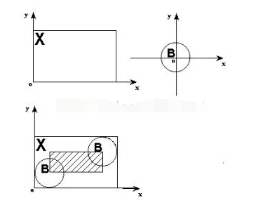2.膨胀     膨胀(dilation)可以看做是腐蚀的对偶运算，其定义是：把结构元素B平移a后得到Ba，若Ba击中X，我们记下这个a点。所有满足上述条件的a点组成的集合称做X被B膨胀的结果。用公式表示为：D(X)={a | Ba↑X}=X B，如图6.13所示。图6.13中X是被处理的对象，B是结构元素，不难知道，对于任意一个在阴影部分的点a，Ba击中X，所以X被B膨胀的结果就是那个阴影部分。阴影部分包括X的所有范围，就象X膨胀了一圈似的，这就是为什么叫膨胀的原因。原理图如下：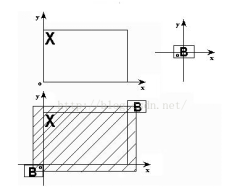3.击中与击不中原理     击中击不中变换(HMT)需要两个结构元素B1和B2,合成一个结构元素对B=(B1,B2)一个用于探测图像内部,作为击中部分;另一个用于探测图像外部,作为击不中部分。显然,B1和B2是不应该相连接的,即B1∩B2=Φ。击中击不中变换的数学表达式为： g(x, y)=hitmiss[f(x, y), B]=erode[f(x, y), B1]AND erode[fc(x, y), B2] 其中,fc(x,y)表示的是f(x,y)的补集。
Hit-miss算法步骤： 击中击不中变换是形态学中用来检测特定形状所处位置的一个基本工具。它的原理就是使用腐蚀；如果要在一幅图像A上找到B形状的目标，我们要做的是：
建立一个比B大的模板W；使用此模板对图像A进行腐蚀，得到图像假设为Process1;用B减去W，从而得到V模板(W-B)；使用V模板对图像A的补集进行腐蚀，得到图像假设为Process2Process1与Process2取交集；得到的结果就是B的位置。这里的位置可能不是B的中心位置，要视W-B时对齐的位置而异； 其实很简单，两次腐蚀，然后交集，结果就出来了。
六、实验过程及代码
1.去除噪声、空洞
A1=imread('noise.png');

C=[0 1 0
1 1 1
0 1 0];
A2=imdilate(A1,C);%图像A1被结构元素B膨胀
A3=imdilate(A2,C);
A4=imdilate(A3,C);
A5=imdilate(A4,C);
A6=imdilate(A5,C);
A7=imdilate(A6,C);
A8=imdilate(A7,C);

A9=imerode(A8,C); %腐蚀
A10=imerode(A9,C);
A11=imerode(A10,C);
A12=imerode(A11,C);
A13=imerode(A12,C);
A14=imerode(A13,C);
A15=imerode(A14,C);
A16=imerode(A15,C);
A17=imerode(A16,C);
A18=imerode(A17,C);
A19=imerode(A18,C);
A20=imerode(A19,C);

D=strel('rectangle',[2 2]);
A21=imdilate(A20,C);
A22=imdilate(A21,C);
A23=imdilate(A22,C);
A24=imdilate(A23,C);
A25=imdilate(A24,C);
A26=imdilate(A25,C);
A27=imdilate(A26,C);

imshow(A27);

2.击中与击不中
t=zeros(500,500);

for i=100:200
for j=100:200
t(i,j)=1;
end
end

for i=70:140
for j=370:440
t(i,j)=1;
end
end

for i=300:400
for j=300:480
t(i,j)=1;
end
end

imshow(t);%构造原图

m=zeros(100,100);

for i=1:100
for j=1:100
m(i,j)=1;
end
end

figure,imshow(m);%构造m结构元素

n=zeros(120,120);

for i=1:120
for j=1:120
n(i,j)=1;
end
end

for i=10:110
for j=10:110
n(i,j)=0;
end
end

figure,imshow(n);%构造n结构元素 与m交集为空

x1=imerode(t,m);
figure,imshow(x1);%原图被m结构元素腐蚀后
x2=imerode(~t,n);
figure,imshow(x2);%原图补集被n结构元素腐蚀后

x3=x1.*x2;
figure,imshow(x3);%求两张腐蚀后图像的交集

七、实验结果与分析
1.去除噪声、空洞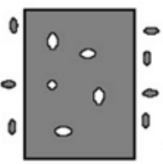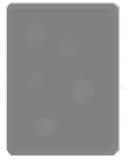图 1 左：原图 右：处理后
2.击中与击不中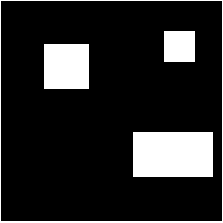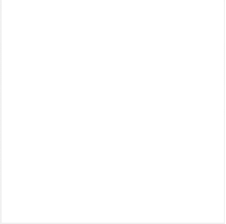图 2原图(左) 结构元素m(右，纯白色)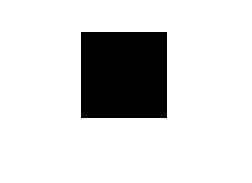图 3 原图补集(左) n结构元素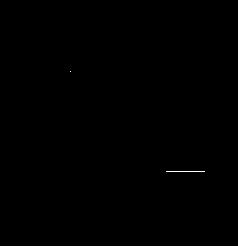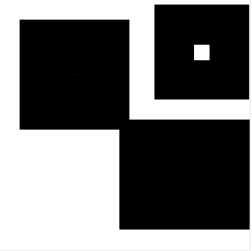图 4 m对原图腐蚀后(左) n对原图补集腐蚀后(右)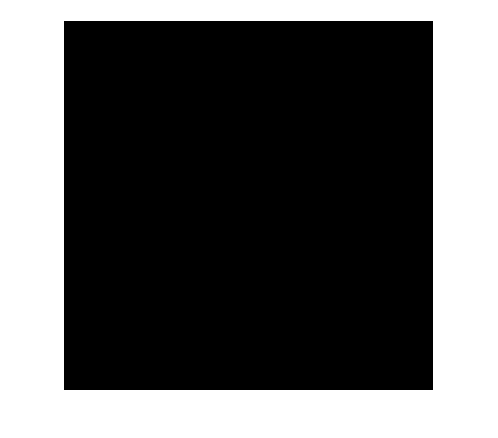图 5两腐蚀图求交集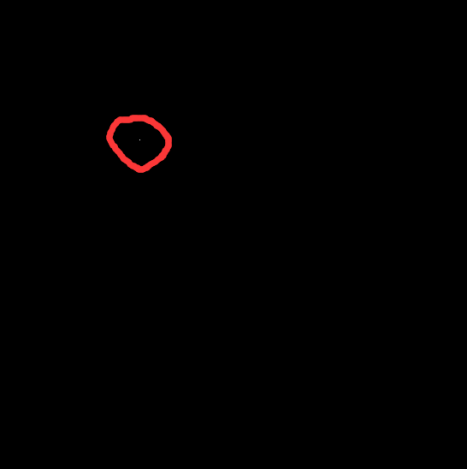图 6 最终结果图（红色线圈出的位置就是我们需要找的位置）
八、实验总结及心得体会
在这次实验中，自己学会了数字图像处理中的腐蚀、膨胀操作，其原理就是利用集合论的知识，对图像进行处理。这利用MATLAB编程的过程中，遇到了一些小困难，主要原因还是对一些函数的不熟悉。在击中与击不中这一块，感触颇多。这一块内容几乎用了半天的时间，才搞懂。这里暴露出的问题时原理理解的不是很深刻，加之MATLAB这里与课本上的例子有点出入，在这里饶了一点弯路。其实还好，在csdn上看别人写的文章，多看几遍，照着例子多看几遍，最后还是明白了。在击中与击不中实验中，我采用的方法是先自己创造出原图、两个结构元素，这样是为了更好的和课本一致，然后稍微改动了一些，感觉课本在讲这块有点错误，课本是从原理角度解释击中与击不中，在MATLAB中，我们则需要取反即可。
更多
获取更多资料、代码，微信公众号：海轰Pro 回复 海轰 即可
展开全文MATLAB 数字图像处理
• 提出了一种基于数学形态学滤波的多分辨力图像融合。这种融合方法使用了形态学开闭运算构造了低通与高通滤波器, 将...融合实验表明, 该方法优于传统的形态学金字塔图像融合, 衬比度金字塔图像融合和小波分解图像融合。
• 第 4讲 :形态实验学课程 形态实验学课程 启发与创新思维 如何启发学生的创新思维 第一培养兴趣 第二丰富的知识 * * 兴趣是创新的动力和先导一个人的创造性成果无一不是在对所研究的问题产生浓厚兴趣的情况下所取得的...
• 针对已有的数学形态学边缘检测算法对彩色噪声图像检测到的彩色边缘信息不够完整、清晰，提出了一种基于HSI色彩空间的多尺度多结构元的数学形态学边缘检测算法，采用以尺度和结构两个单位元素进行横向和纵向的拓展，...HSI色彩空间 边缘检测...